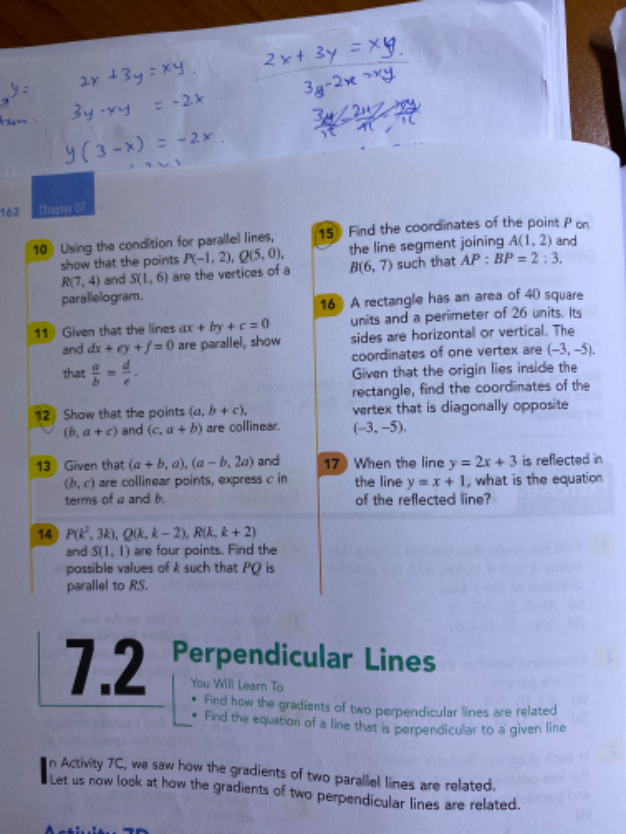# QuestionKindly help me with q11&15 thanks

Solution for 11

ax+by + c = 0                        dx+ey + f = 0

by = -ax -c                              ey = -dx -f

y = -(a/b)x – c/b                    y = -(d/e)x – f/e

When 2 lines are parallel, their gradients are equal,

so   -(a/b) = -(d/e)    =>  (a/b) = (d/e)   (proven)

Solution for 16

A = (1, 2)

B = (6,7)

AP : BP  = 2 : 3

The coordinates of the point P(x, y) which divides the line segment joining the points A (x₁, y₁) and B(x₂, y₂), internally, in the ratio m₁ : m₂ is given by the section formula:

P (x, y) = [(mx₂ + nx₁)/ (m + n) , (my₂ + ny₁)/(m + n) ]

= {  [(2×6) + (3×1)]  / (2+3) ,  { [(2×7) + (3×2)] / (2+3) }

= [ (12+3) / 5 ,  (14+6) / 5 ]

= [ 15/5 , 20/5]

=(3 , 4)

Hence the coordinates of P is (3 , 4).